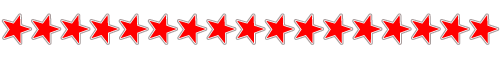Like   Tweet   Pin   +1   in
 table div table+table div table{width:100%;padding:0}table div table+table div table table{padding:0;float:left!important;width:51.886%!important}table div table+table div table table+table td,table div table+table div table td{padding-left:0;padding-right:0}table div table+table div table table td{padding-left:0;padding-right:20px}table div table+table div table table+table{float:left!important;width:48.114%!important}/* styles */
 table div table+table+table div table{width:100%;padding:0}table div table+table+table div table table{padding:0;float:left!important;width:51.886%!important}table div table+table+table div table table+table td,table div table+table+table div table td{padding-left:0;padding-right:0}table div table+table+table div table table td{padding-left:0;padding-right:20px}table div table+table+table div table table+table{float:left!important;width:48.114%!important}/* styles */
 table div table+table+table+table div table{width:100%;padding:0}table div table+table+table+table div table table{padding:0;float:left!important;width:51.886%!important}table div table+table+table+table div table table+table td,table div table+table+table+table div table td{padding-left:0;padding-right:0}table div table+table+table+table div table table td{padding-left:0;padding-right:20px}table div table+table+table+table div table table+table{float:left!important;width:48.114%!important}/* styles */
 table div table+table+table+table+table div table{width:100%;padding:0}table div table+table+table+table+table div table table{padding:0;float:left!important;width:51.886%!important}table div table+table+table+table+table div table table+table td,table div table+table+table+table+table div table td{padding-left:0;padding-right:0}table div table+table+table+table+table div table table td{padding-left:0;padding-right:20px}table div table+table+table+table+table div table table+table{float:left!important;width:48.114%!important}/* styles */
 table div table+table+table+table+table+table div table{width:100%;padding:0}table div table+table+table+table+table+table div table table{padding:0;float:left!important;width:51.886%!important}table div table+table+table+table+table+table div table table+table td,table div table+table+table+table+table+table div table td{padding-left:0;padding-right:0}table div table+table+table+table+table+table div table table td{padding-left:0;padding-right:20px}table div table+table+table+table+table+table div table table+table{float:left!important;width:48.114%!important}/* styles */
 table div table+table+table+table+table+table+table div table{width:100%;padding:0}table div table+table+table+table+table+table+table div table table{padding:0;float:left!important;width:51.886%!important}table div table+table+table+table+table+table+table div table table+table td,table div table+table+table+table+table+table+table div table td{padding-left:0;padding-right:0}table div table+table+table+table+table+table+table div table table td{padding-left:0;padding-right:20px}table div table+table+table+table+table+table+table div table table+table{float:left!important;width:48.114%!important}/* styles */
 table div table+table+table+table+table+table+table+table div table{width:100%;padding:0}table div table+table+table+table+table+table+table+table div table table{padding:0;float:left!important;width:50.754%!important}table div table+table+table+table+table+table+table+table div table table+table td,table div table+table+table+table+table+table+table+table div table td{padding-left:0;padding-right:0}table div table+table+table+table+table+table+table+table div table table td{padding-left:0;padding-right:20px}table div table+table+table+table+table+table+table+table div table table+table{float:left!important;width:49.246%!important}/* styles */
 table div table+table+table+table+table+table+table+table+table div table{width:100%;padding:0}table div table+table+table+table+table+table+table+table+table div table table{padding:0;float:left!important;width:51.886%!important}table div table+table+table+table+table+table+table+table+table div table table+table td,table div table+table+table+table+table+table+table+table+table div table td{padding-left:0;padding-right:0}table div table+table+table+table+table+table+table+table+table div table table td{padding-left:0;padding-right:20px}table div table+table+table+table+table+table+table+table+table div table table+table{float:left!important;width:48.114%!important}/* styles */
 table div table+table+table+table+table+table+table+table+table+table div table{width:100%;padding:0}table div table+table+table+table+table+table+table+table+table+table div table table{padding:0;float:left!important;width:51.886%!important}table div table+table+table+table+table+table+table+table+table+table div table table+table td,table div table+table+table+table+table+table+table+table+table+table div table td{padding-left:0;padding-right:0}table div table+table+table+table+table+table+table+table+table+table div table table td{padding-left:0;padding-right:20px}table div table+table+table+table+table+table+table+table+table+table div table table+table{float:left!important;width:48.114%!important}/* styles */Reach out to promoters and beyond with your message, photos and videos via Raestar's Promotions | Danielle White +44 (0)7841 675 263 | daniraestar@aol.com | www.daniraestar.com #BLACKLIVESMATTER
JAZZ ALBUM SHOWCASE